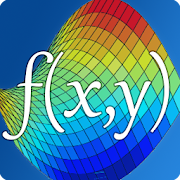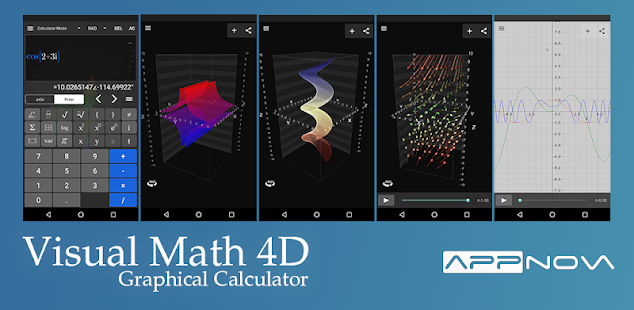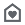# Visual Math 4DEveryone
913
Offers in-app purchasesVisual Math 4D is a graphical calculator that allows you to visualize and solve your mathematical equations. It supports various types of equations, such as spherical, parametrical, polar and cartesian and implicit equations, which can be visualized and animated in 2D and 3D. You can also plot and animate vector fields in 2D and 3D.

Features:
- solve equations and visualize their intersections
- plot cartesian functions with intersections
- plot polar- and spherical functions
- plot parametrical equations
- plot complex functions (draw real part & imaginary part)
- plot vector fields in 2D and 3D
- plot implicit equations in 2D and 3D
- plot contours of your functions
- complex numbers
- vectors & matrices
- truth- and value tables
- trigonometrical and hyperbolic functions
- piecewise-defined functions
- logarithmic functions
- logical & binary operators
- definite integral
- n-th derivations
- statistical functions
- physical and mathematical constants with units
- animate variables
- share content with other apps
- no network connection required
- and a lot more...

The app is easy to use and helps students and engineers to visualize and solve their mathematical equations.
Collapse

## Reviews

Review policy and info
4.5
913 total
5
4
3
2
1

## What's New

Updated contour plot and some bugfixes
Collapse

Eligible for Family LibraryLearn More
Updated
February 26, 2019
Size
22M
Installs
100,000+
Current Version
5.3.1
Requires Android
5.0 and up
Content Rating
Everyone
In-app Products
\$0.99 - \$3.99 per item
Permissions
Offered By
appnova
Developer
Ernststraße 59 13509 Berlin